图像预处理_图像预处理流程 - CSDN

• 图像预处理的主要目的是消除图像中无关的信息，恢复有用的真实信息，增强有关信息的可检测性、最大限度地简化数据，从而改进特征提取、图像分割、匹配和识别的可靠性。一般的预处理流程为：灰度化->几何变换->...
图像分析中，图像质量的好坏直接影响识别算法的设计与效果的精度，因此在图像分析（特征提取、分割、匹配和识别等）前，需要进行预处理。图像预处理的主要目的是消除图像中无关的信息，恢复有用的真实信息，增强有关信息的可检测性、最大限度地简化数据，从而改进特征提取、图像分割、匹配和识别的可靠性。一般的预处理流程为：灰度化->几何变换->图像增强

一、灰度化

对彩色图像进行处理时，我们往往需要对三个通道依次进行处理，时间开销将会很大。因此，为了达到提高整个应用系统的处理速度的目的，需要对彩色图像进行灰度化以减少所需处理的数据量。
在RGB模型中，如果R=G=B时，则彩色表示一种灰度颜色，其中R=G=B的值叫灰度值，因此，灰度图像每个像素只需一个字节存放灰度值（又称强度值、亮度值），灰度范围为0-255。一般有分量法、最大值法、平均值法、加权平均法四种方法对彩色图像进行灰度化。

加权平均法

根据重要性及其它指标，将三个分量以不同的权值进行加权平均。由于人眼对绿色的敏感最高，对蓝色敏感最低，因此，按下式对RGB三分量进行加权平均能得到较合理的灰度图像。

转换算法 L = R * 299/1000 + G * 587/1000+ B * 114/1000

二、几何变换

图像几何变换又称为图像空间变换，通过平移、转置、镜像、旋转、缩放等几何变换对采集的图像进行处理，用于改正图像采集系统的系统误差和仪器位置（成像角度、透视关系乃至镜头自身原因）的随机误差。此外，还需要使用灰度插值算法，因为按照这种变换关系进行计算，输出图像的像素可能被映射到输入图像的非整数坐标上。通常采用的方法有最近邻插值、双线性插值和双三次插值。

1、最近邻插值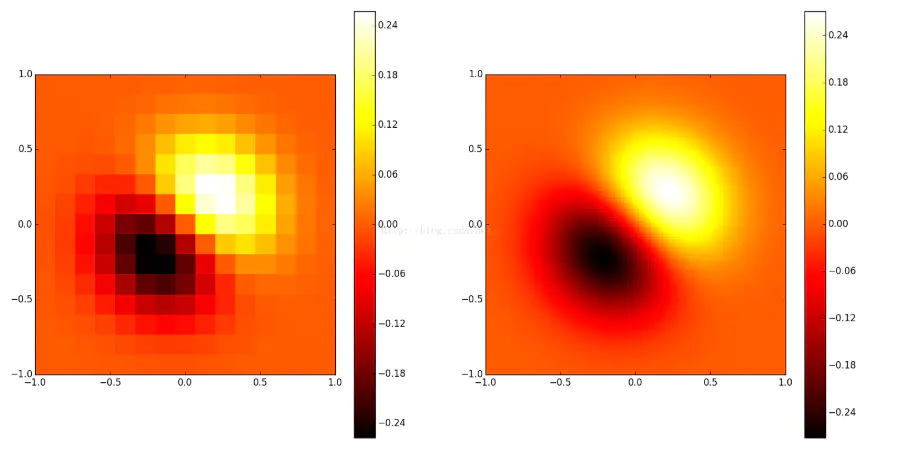# coding = utf-8
"""
最近邻插值图像缩放演示
"""
import numpy as np
from scipy import interpolate
import pylab as pl
import matplotlib as mpl

def func(x, y):
return (x+y)*np.exp(-5.0*(x**2 + y**2))

# X-Y轴分为15*15的网格
y,x= np.mgrid[-1:1:15j, -1:1:15j]

fvals = func(x,y) # 计算每个网格点上的函数值  15*15的值

#三次样条二维插值
newfunc = interpolate.interp2d(x, y, fvals, kind='cubic')

# 计算100*100的网格上的插值
xnew = np.linspace(-1,1,100)#x
ynew = np.linspace(-1,1,100)#y
fnew = newfunc(xnew, ynew)#仅仅是y值   100*100的值

# 绘图
# 为了更明显地比较插值前后的区别，使用关键字参数interpolation='nearest'
# 关闭imshow()内置的插值运算。
pl.subplot(121)
im1=pl.imshow(fvals, extent=[-1,1,-1,1], cmap=mpl.cm.hot, interpolation='nearest', origin="lower")#pl.cm.jet
pl.colorbar(im1)

pl.subplot(122)
im2=pl.imshow(fnew, extent=[-1,1,-1,1], cmap=mpl.cm.hot, interpolation='nearest', origin="lower")
pl.colorbar(im2)
pl.show()

2、双线性插值

# encoding: utf-8
'''
双线性插值图像缩放算法
'''
import numpy as np
import cv2 as cv
import math

def bi_linear(src, dst, target_size):
th, tw = target_size, target_size
emptyImage = np.zeros(target_size, np.uint8)
for k in range(3):
for i in range(th):
for j in range(tw):
# 首先找到在原图中对应的点的(X, Y)坐标
corr_x = (i+0.5)/th*pic.shape-0.5
corr_y = (j+0.5)/tw*pic.shape-0.5
point1 = (math.floor(corr_x), math.floor(corr_y))   # 左上角的点
point2 = (point1, point1+1)
point3 = (point1+1, point1)
point4 = (point1+1, point1+1)

fr1 = (point2-corr_y)*pic[point1, point1, k] + (corr_y-point1)*pic[point2, point2, k]
fr2 = (point2-corr_y)*pic[point3, point3, k] + (corr_y-point1)*pic[point4, point4, k]
emptyImage[i, j, k] = (point3-corr_x)*fr1 + (corr_x-point1)*fr2

cv.imwrite(dst, emptyImage)

def main():
src = 'pic/raw_1.jpg'
dst = 'pic/new_1.png'
target_size = (300, 200, 3)     # 变换后的图像大小

bi_linear(src, dst, target_size)

if __name__ == '__main__':
main()

三、图像增强

增强图像中的有用信息，它可以是一个失真的过程，其目的是要改善图像的视觉效果，针对给定图像的应用场合，有目的地强调图像的整体或局部特性，将原来不清晰的图像变得清晰或强调某些感兴趣的特征，扩大图像中不同物体特征之间的差别，抑制不感兴趣的特征，使之改善图像质量、丰富信息量，加强图像判读和识别效果，满足某些特殊分析的需要。
图像增强可分成两大类：频率域法和空间域法。

1、频率域法

我们知道声音的频率是什么意思。高频是指音调很高的噪音，例如鸟的唧唧声或小提琴的声音。低频是指音调很低的声音，例如深沉的声音或低音鼓。对于声音来说，频率是指声波的振荡速度；振荡通常以周期 (Hz) 表示，高音调由高频声波组成。低频和高频声波的示例如下图所示。在 y 轴上是振幅，即声压相对于声音感知音量的测量结果；x 轴是时间。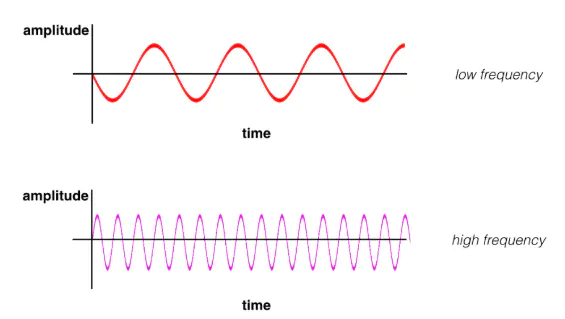同样，图像中的频率是指变化速度。但是图像的变化是什么意思？图像会在空间中变化，高频图像是指强度变化很多的图像。亮度水平从一个像素到另一个像素变化很快。低频图像可能是亮度比较均匀或变化很慢的图像。大多数图像同时具有高频和低频部分。在上图中，丝巾和条纹衬衫部分是高频图像模式；此部分非常快速地从一个亮度变成另一个亮度。在同一图像的更上方，我们看到天空和背景变化很缓慢，称为平滑的低频模式。
频率域法是一种间接图像增强算法，把图像看成一种二维信号，对其进行基于二维傅里叶变换的信号增强。采用低通滤波（即只让低频信号通过）法，可去掉图中的噪声；采用高通滤波法，则可增强边缘等高频信号，使模糊的图片变得清晰。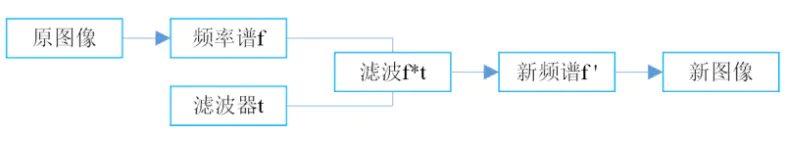图像的傅里叶变换
进行傅立叶变换（FT）后，可以显示图像的频率分量。

# coding = utf-8
import numpy as np
import matplotlib.pyplot as plt
import cv2 as cv

def ft_image(norm_image):
'''
二维傅里叶变换，接收一个灰度化且归一化的图像返回图像的频谱变换图
'''
f = np.fft.fft2(norm_image)
fshift = np.fft.fftshift(f)
frequency_tx = 20*np.log(np.abs(fshift))

return frequency_tx

# 图像读取并灰度化
image_stripes = cv.cvtColor(image_stripes, cv.COLOR_BGR2RGB)
gray_stripes = cv.cvtColor(image_stripes, cv.COLOR_RGB2GRAY)

image_solid = cv.cvtColor(image_solid, cv.COLOR_BGR2RGB)
gray_solid = cv.cvtColor(image_solid, cv.COLOR_RGB2GRAY)

# 为了便于后续处理将颜色空间从 [0,255] 归一化到 [0,1]
norm_stripes = gray_stripes/255.0
norm_solid = gray_solid/255.0

# 生成频谱图
f_stripes = ft_image(norm_stripes)
f_solid = ft_image(norm_solid)

# 可视化
f, (ax1,ax2,ax3,ax4) = plt.subplots(1, 4, figsize=(20,10))

ax1.set_title('original image')
ax1.imshow(image_stripes)
ax2.set_title('frequency transform image')
ax2.imshow(f_stripes, cmap='gray')

ax3.set_title('original image')
ax3.imshow(image_solid)
ax4.set_title('frequency transform image')
ax4.imshow(f_solid, cmap='gray')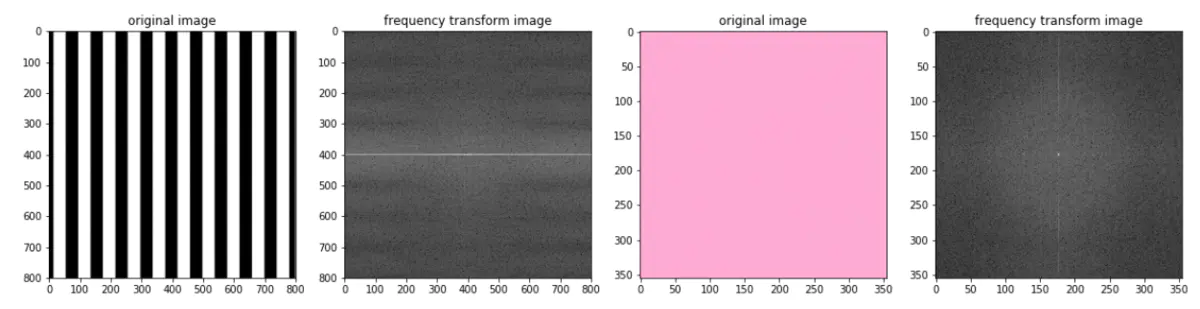图像处理与二维傅里叶变换
二维频谱中的每一个点都是一个与之一一对应的二维正弦/余弦波。拓展阅读

（1）理想的高/低通滤波

顾名思义，低通滤波器为：让低频信息通过，过滤高频信息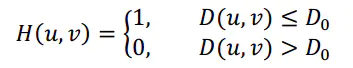其中，D0表示通带半径，D(u，v)是到频谱中心的距离（欧式距离），计算公式如下：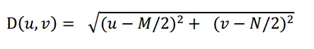M和N表示频谱图像的大小，（M/2，N/2）即为频谱中心
tips：高通滤波器与此相反，1减去低通滤波模板即可

def make_transform_matrix(image_arr, d0, ftype):
'''
构建理想高/低通滤波器
INPUT  -> 图像数组, 通带半径, 类型
'''
transfor_matrix = np.zeros(image_arr.shape, dtype=np.float32)  # 构建滤波器
w, h = transfor_matrix.shape
for i in range(w):
for j in range(h):
distance = np.sqrt((i - w/2)**2 + (j - h/2)**2)
if distance < d0:
transfor_matrix[i, j] = 1
else:
transfor_matrix[i, j] = 0
if ftype == 'low':
return transfor_matrix
elif ftype == 'high':
return 1 - transfor_matrix

# 图像灰度化
img_arr = np.array(Image.open('33.jpg').convert('L'))

# 将图像从空间域转换到频率域
f = np.fft.fft2(img_arr)
fshift = np.fft.fftshift(f)

# 生成低通滤波器
F_filter1 = make_transform_matrix(img_arr, 30, 'low')
# 滤波
result = fshift*F_filter1
# 将图像从频率域转换到空间域
img_d1 = np.abs(np.fft.ifft2(np.fft.ifftshift(result)))

# 可视化
plt.imshow(array_to_image(img_d1))
plt.show()

（2）高斯高/低通滤波

Guassian低通滤波器函数为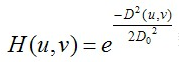tips： 1减去低通滤波模板即可得到高通滤波模板

def make_transform_matrix(image_arr, d0, ftype='low'):
'''
构建高斯高/低通滤波
INPUT  -> 图像数组, 通带半径, 类型
'''
transfor_matrix = np.zeros(image_arr.shape, dtype=np.float32)  # 构建滤波器
w, h = image_arr.shape
for i in range(w):
for j in range(h):
distance = np.sqrt((i - w/2)**2 + (j - h/2)**2)
transfor_matrix[i, j] = np.e ** (-1 * (distance ** 2 / (2 * d0 ** 2)))  # Gaussian滤波函数
if ftype == 'low':
return transfor_matrix
elif ftype == 'high':
return 1 - transfor_matrix

# 图像灰度化
img_arr = np.array(Image.open('33.jpg').convert('L'))

# 将图像从空间域转换到频率域
f = np.fft.fft2(img_arr)
fshift = np.fft.fftshift(f)

# 生成低通滤波器
F_filter1 = make_transform_matrix(img_arr, 30, 'low')
# 滤波
result = fshift*F_filter1
# 将图像从频率域转换到空间域
img_d1 = np.abs(np.fft.ifft2(np.fft.ifftshift(result)))

# 可视化
plt.imshow(array_to_image(img_d1))
plt.show()

2、空间域法

空间域法是一种直接图像增强算法，分为点运算算法和邻域去噪算法。
点运算算法即灰度变换（伽马变换、对数增强）和直方图修正等。
邻域增强算法分为图像平滑和锐化两种。平滑常用算法有均值滤波、中值滤波。锐化常用算法有梯度法（如Roberts梯度法）、算子法（Sobel算子和拉普拉斯算子等）、掩模匹配法、统计差值法等。

（1）点运算算法

from skimage import exposure

def image_gamma_transform(pil_im, gamma):
'''
伽马变换
INPUT  -> 单张图文件
OUTPUT -> 处理后的图文件
'''
image_arr = np.array(pil_im)
return array_to_image(image_arr2)

def image_gamma_transform2(pil_im, gamma):
'''
伽马变换2（源码实现）
INPUT  -> 单张图文件
OUTPUT -> 处理后的图文件
'''
image_arr = np.array(pil_im)
image_arr2 = np.power(image_arr / float(np.max(image_arr)), gamma)
return array_to_image(image_arr2*float(np.max(image_arr)))

def image_log_transform(pil_im):
'''
图像对数增强
INPUT  -> 单张图文件
OUTPUT -> 处理后的图文件
'''
image_arr = image_to_array(pil_im)
if len(image_arr.shape) == 3:
for i in range(3):
image_arr[:,:,i] = 255/np.log(255+1)*np.log(1+image_arr[:,:,i])
return array_to_image(image_arr)
elif len(image_arr.shape) == 2:
# image_arr = 255/np.log(np.max(image_arr)+1)*np.log(1+image_arr)
image_arr = 255/np.log(255+1)*np.log(1+image_arr)
return array_to_image(image_arr)

def image_histeq(pil_im):
'''
直方图均衡化
INPUT  -> 单张图文件
OUTPUT -> 处理后的图文件
'''
# 计算图像的直方图
image_arr = image_to_array(pil_im)
imhist, bins = np.histogram(image_arr.flatten(), 256, normed=True)
cdf = imhist.cumsum()   # 累计分布函数
cdf = 255*cdf/cdf[-1]   # 归一化
# 使用累计分布函数的线性插值计算新的像素值
image_arr2 = np.interp(image_arr.flatten(), bins[:-1], cdf)
return array_to_image(image_arr2.reshape(image_arr.shape))

（2）邻域增强算法之平滑

均值滤波

也称线性滤波，主要采用邻域平均法。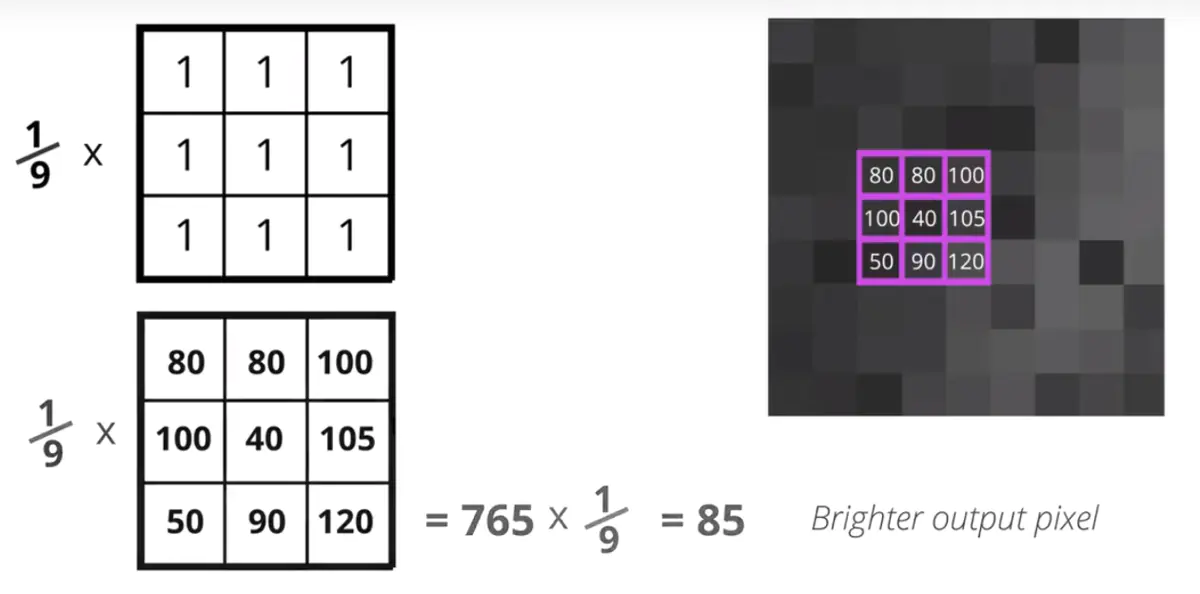均值滤波的核心思想是其将整个图像看成是由很多灰度恒定的小块组成，相邻像素间相关性很强（任意一点的像素值，都是周围N*M个像素值的均值），但噪声具有统计独立性。通过均值滤波减少了灰度的突变。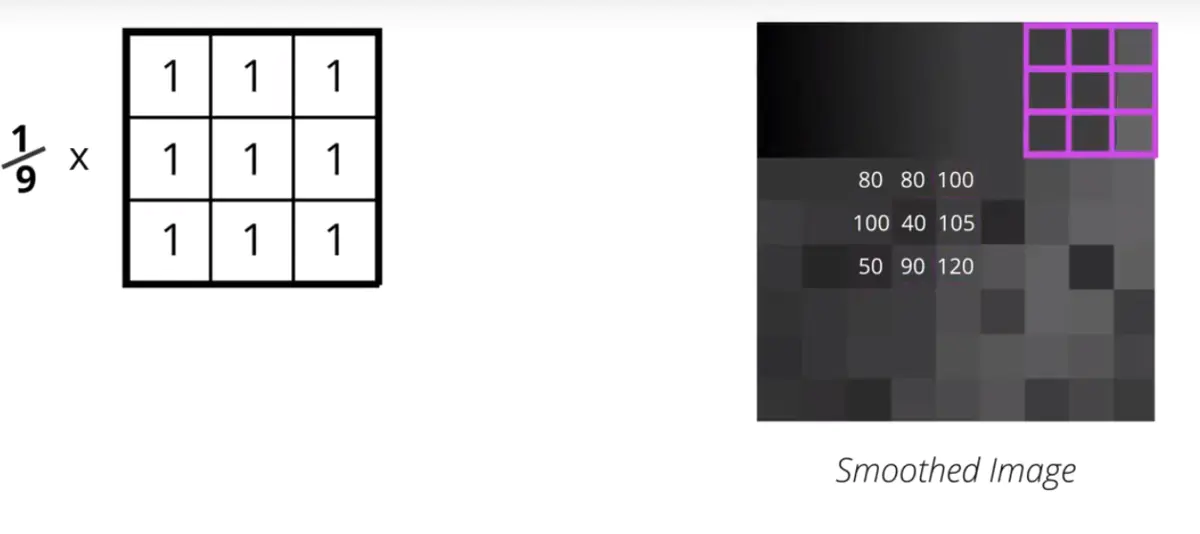均值滤波可以加上两个参数，即迭代次数，Kernel数据大小。一个相同的Kernel，但是多次迭代就会效果越来越好。同样，迭代次数相同，Kernel矩阵越大，均值滤波的效果就越明显。

from scipy.signal import convolve2d

def image_mean_filter(pil_im):
'''
均值滤波
INPUT  -> 单张图文件
OUTPUT -> 处理后的图文件
'''
image_arr = image_to_array(pil_im)
dst_arr = np.zeros_like(image_arr)
# 卷积核-均值算子
mean_operator = np.array([[1, 1, 1],
[1, 1, 1],
[1, 1, 1]])/9
if len(image_arr.shape) == 3:
for i in range(3):
dst_arr[:,:,i] = convolve2d(image_arr[:,:,i], mean_operator, mode="same")
elif len(image_arr.shape) == 2:
dst_arr = convolve2d(image_arr, mean_operator, mode="same")

return array_to_image(dst_arr)

中值滤波

中值滤波也是消除图像噪声最常见的手段之一，特别是消除椒盐噪声，中值滤波的效果要比均值滤波更好。中值滤波是跟均值滤波唯一不同是，不是用均值来替换中心每个像素，而是将周围像素和中心像素排序以后，取中值。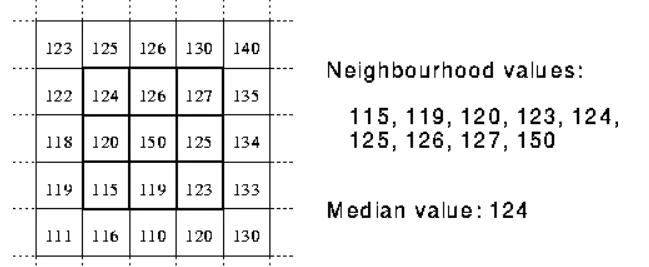from scipy.ndimage import filters

def image_medium_filter(image_arr, K=5):
'''
中值滤波
中值平滑只对特别尖锐的信号平滑
INPUT  -> 图像数组
OUTPUT -> 去噪后的图像数组
'''
if len(image_arr.shape) == 3:
for i in range(3):
image_arr[:,:,i] = filters.median_filter(image_arr[:,:,i], K)
return image_arr
elif len(image_arr.shape) == 2:
image_arr = filters.medianBlur(image_arr, K)
return image_arr

（3）邻域增强算法之锐化

from scipy.signal import convolve2d

def image_laplace_filter(pil_im):
'''
拉普拉斯算子增强
INPUT  -> 单张图文件
OUTPUT -> 处理后的图文件
'''
image_arr = np.array(pil_im)
dst_arr = np.zeros_like(image_arr)
filter_arr = dst_arr
# 卷积核-拉普拉斯算子
laplace_operator = np.array([[0, -1, 0],
[-1, 4, -1],
[0, -1, 0]])
if len(image_arr.shape) == 3:
for i in range(3):
dst_arr[:,:,i] = convolve2d(image_arr[:,:,i], laplace_operator, mode="same")
filter_arr[:,:,i] = image_Gaussian_filter(dst_arr[:,:,i], 5)
elif len(image_arr.shape) == 2:
dst_arr = convolve2d(image_arr, laplace_operator, mode="same")
filter_arr = image_Gaussian_filter(dst_arr, 5)
dst_arr = image_arr + filter_arr
dst_arr = dst_arr / 255.0
# 饱和处理
return array_to_image(dst_arr*255)


展开全文• ## 图像处理之预处理方法

万次阅读 多人点赞 2018-08-28 15:50:34
图像预处理的主要目的是消除图像中无关的信息，恢复有用的真实信息，增强有关信息的可检测性、最大限度地简化数据，从而改进特征提取、图像分割、匹配和识别的可靠性。一般的预处理流程为：1灰度化-&gt;2几何...
图像分析中，图像质量的好坏直接影响识别算法的设计与效果的精度，因此在图像分析（特征提取、分割、匹配和识别等）前，需要进行预处理。图像预处理的主要目的是消除图像中无关的信息，恢复有用的真实信息，增强有关信息的可检测性、最大限度地简化数据，从而改进特征提取、图像分割、匹配和识别的可靠性。一般的预处理流程为：1灰度化->2几何变换->3图像增强

1灰度化

灰度化，在RGB模型中，如果R=G=B时，则彩色表示一种灰度颜色，其中R=G=B的值叫灰度值，因此，灰度图像每个像素只需一个字节存放灰度值（又称强度值、亮度值），灰度范围为0-255。一般有分量法 最大值法平均值法加权平均法四种方法对彩色图像进行灰度化。

对彩色图像进行处理时，我们往往需要对三个通道依次进行处理，时间开销将会很大。因此，为了达到提高整个应用系统的处理速度的目的，需要减少所需处理的数据量。

1.分量法

将彩色图像中的三分量的亮度作为三个灰度图像的灰度值，可根据应用需要选取一种灰度图像。

f1(i,j)=R(i,j)f2(i,j)=G(i,j)f3(i,j)=B(i,j)

其中fk(i,j)(k=1,2,3)为转换后的灰度图像在（i,j）处的灰度值。

彩色图像：彩色图的三分量灰度图：（a）R分量灰度图 （b）G分量灰度图 （c）B分量灰度图

2.最大值法

将彩色图像中的三分量亮度的最大值作为灰度图的灰度值。

f(i,j)=max(R(i,j),G(i,j),B(i,j))

3.平均值法

将彩色图像中的三分量亮度求平均得到一个灰度值。

f(i,j)=(R(i,j)+G(i,j)+B(i,j)) /3

4.加权平均法

根据重要性及其它指标，将三个分量以不同的权值进行加权平均。由于人眼对绿色的敏感最高，对蓝色敏感最低，因此，按下式对RGB三分量进行加权平均能得到较合理的灰度图像。

f(i,j)=0.30R(i,j)+0.59G(i,j)+0.11B(i,j)

2几何变换

图像几何变换又称为图像空间变换，通过平移、转置、镜像、旋转、缩放等几何变换对采集的图像进行处理，用于改正图像采集系统的系统误差和仪器位置（成像角度、透视关系乃至镜头自身原因）的随机误差。此外，还需要使用灰度插值算法，因为按照这种变换关系进行计算，输出图像的像素可能被映射到输入图像的非整数坐标上。通常采用的方法有最近邻插值、双线性插值和双三次插值。

3图像增强

增强图像中的有用信息，它可以是一个失真的过程，其目的是要改善图像的视觉效果，针对给定图像的应用场合，有目的地强调图像的整体或局部特性，将原来不清晰的图像变得清晰或强调某些感兴趣的特征，扩大图像中不同物体特征之间的差别，抑制不感兴趣的特征，使之改善图像质量、丰富信息量，加强图像判读和识别效果，满足某些特殊分析的需要。图像增强算法可分成两大类：空间域法和频率域法。

3.1空间域法

空间域法是一种直接图像增强算法，分为点运算算法和邻域去噪算法。点运算算法即灰度级校正、灰度变换（又叫对比度拉伸）和直方图修正等。邻域增强算法分为图像平滑和锐化两种。平滑常用算法有均值滤波、中值滤波、空域滤波。锐化常用算法有梯度算子法、二阶导数算子法、高通滤波、掩模匹配法等。

3.2频率域法

频率域法是一种间接图像增强算法，常用的频域增强方法有低通滤波器和高通滤波器。低频滤波器有理想低通滤波器、巴特沃斯低通滤波器、高斯低通滤波器、指数滤波器等。高通滤波器有理想高通滤波器、巴特沃斯高通滤波器、高斯高通滤波器、指数滤波器。

图像增强可分成两大类：频率域法和空间域法。

前者把图像看成一种二维信号，对其进行基于二维傅里叶变换的信号增强。采用低通滤波（即只让低频信号通过）法，可去掉图中的噪声；采用高通滤波法，则可增强边缘等高频信号，使模糊的图片变得清晰。

后者空间域法中具有代表性的算法有局部求平均值法和中值滤波（取局部邻域中的中间像素值）法等，它们可用于去除或减弱噪声。

方法

图像增强的方法是通过一定手段对原图像附加一些信息或变换数据，有选择地突出图像中感兴趣的特征或者抑制(掩盖)图像中某些不需要的特征，使图像与视觉响应特性相匹配。

在图像增强过程中，不分析图像降质的原因，处理后的图像不一定逼近原始图像。图像增强技术根据增强处理过程所在的空间不同，可分为基于空域的算法和基于频域的算法两大类。

空域法是对图像中的像素点进行操作，用公式描述如下：

g(x,y)=f(x,y)*h(x,y)

其中是f(x,y)是原图像；h(x,y)为空间转换函数；g(x,y)表示进行处理后的图像。

基于空域的算法处理时直接对图像灰度级做运算，基于频域的算法是在图像的某种变换域内对图像的变换系数值进行某种修正，是一种间接增强的算法。

基于空域的算法分为点运算算法   和邻域去噪算法   。

点运算算法即灰度级校正、灰度变换和直方图修正等，目的或使图像成像均匀，或扩大图像动态范围，扩展对比度。

邻域增强算法分为图像平滑和锐化两种。

平滑一般用于消除图像噪声，但是也容易引起边缘的模糊。常用算法有均值滤波、中值滤波。锐化的目的在于突出物体的边缘轮廓，便于目标识别。常用算法有梯度法、算子、高通滤波、掩模匹配法、统计差值法等。


展开全文• 图像预处理 1. 像素亮度变换 像素亮度变换修改像素的亮度，变换只取决与各像素自身的性质。 1.1 位置相关的亮度校正 在理想的情况下，图像获取和数字化设备的灵敏度不应该与图像的位置有关，实际情况下是...
 图像预处理
#1. 像素亮度变换
像素亮度变换修改像素的亮度，变换只取决与各像素自身的性质。
##1.1 位置相关的亮度校正
在理想的情况下，图像获取和数字化设备的灵敏度不应该与图像的位置有关，实际情况下是不对的。光线离光轴越远透镜对它削弱越多。
​	如果退化具有系统性，就可以通过亮度校正加以抑制。一个乘性的错误系数$e(i,j)$描述相对于理想的等同传递函数的变化。假设$g(i,j)$是原来没有退化的图像，$f(i,j)$是含有退化的图像 $f(i,j) = e(i,j)g(i,j)$
​	如果抓取到已知亮度的一幅参考图像，最简单的情况是具有不变的亮度c，则可以获得错误系数$e(i,j)$.退化结果是图像$f_c(i,j) $。抑制$g(i,j) = \frac{f(i.j)}{e(i,j)} = \frac{cf(i,j)}{f_c(i,j)}$
​	这种方法隐含的假设了变换的线性。
##1.2 灰度级变换
​	灰度级变换不依赖于像素在图像中的位置。一个变换$\mathcal{T}$，将原来范围$[p_0,p_k]$内的亮度$p$变换为一个新范围$[q_0,q_k]$内的亮度$q$。$q = \mathcal{T}(p)$。
​	常见的灰度级变换：分段线性函数$a$增强了图像在亮度$p_1和p_2$之间的图像对比度。函数$b$被称为作亮度阈值化(brightness thresholding)，结果是黑白(black-and-white)图像。
​	直方图均衡化(histogram equalization)： 创建一幅在整个亮度范围内具有相同分布的亮度图像。直方图均衡化增强了靠近直方图极大值附近的亮度的对比度，减少了极小值附近的对比度。

输入的直方图用$H(p)$表示，输入的灰度级范围是$[p_0,p_k]$，找到一个单调的像素亮度变换$q = \mathcal{T}(p)$使输出的直方图$G(q)$在整个输出亮度范围$[q_0,q_k]$是均匀的。
直方图可以看为离散的概率密度函数。变换$\mathcal{T}$的单调意味着$\sum\limits_{i=0}^k G(q_i) = \sum\limits_{i=0}^k H(p_i)$
假设图像有$N$行和列，那么均衡化的直方图$G(q)$就对应着均衡的概率密度函数$f$，其函数值是一个常数$f=\frac{N^2}{q_k-q_0}$
连续密度函数：$N^2\int_{q_0}^q\frac{1}{q_k-q_0}ds=\frac{N^2(q-q_0)}{q_k-q_0}=\int_{p_0}^pH(s)ds \rightarrow q=\mathcal{T}(p)=\frac{q_k-q_0}{N^2}\int_{p_0}^pH(s)ds+q_0$
离散的情况：$q = \mathcal{T}(p)=\frac{q_k-q_0}{N^2}\sum\limits_{i=p_0}^p H(s)+q_0$

算法：直方图均衡化：

对于有$G$个灰度级（一般256）大小为$M\times N$的图像，创建一个长为$G$的数组H并初始化为0。
形成图像直方图：扫描每个像素，增加相应的H成员，当像素$p$具有亮度$g_p$时，做$H[g_p]=H[g_p]+1$。
形成累积的直方图$H_c$： $H_c = H\quad H_c[p] = H_c[p-1]+H[p],p=1,2,\cdots,G-1$
置$T[p]=round(\frac{G-1}{MN}H_c[p])$（这一步骤构造一个是NM倍数的与单调增加的$H_c$中的值对应的查找表，有助于提高实现的效率）。
重新扫描图像，写一个具有灰度级$g_q$的输出图像，设置$g_q = T[g_q]$。

#2 几何变换
​	几何变换是一个矢量函数$T$，将一个像素$(x,y)$映射到一 个新位置$(x^{'},y^{'})$。T定义了两个分量公式$x^{'} = T_x(x,y)\quad y^{'}=T_y(x,y)$
步骤：

像素坐标变换(pixel co-ordinate transformation)
确定亮度值。通常用邻域中的几个点的亮度插值(interpolation)计算的。

##2.1 像素坐标变换
​	坐标：通常用多项式公式来近似$x^{'}=\sum\limits_{r =0}^m\sum\limits_{k=0}^{m-r}a_{rk}x^ry^k\quad y^{'} = \sum\limits_{r =0}^m\sum\limits_{k =0}^{m-r} b_{rk}x^ry^k$
这个变换对于系数$a_{rk},b_{rk}$来说是线性的，因此如果已知在两幅图像中的对应点对$(x,y),(x^{'},y^{'})$，就可以通过求解线性方程组的方程确定$a_{rk},b_{rk}$，一般使用的点数超过系数以便保证稳定性，常使用均方方法(mean square method)
​	在几何变换依赖图像中位置的变化并不快的情况下，使用低阶数的多项式近似，m=2或m=3，至少需要6或10个对应点对。对应点在图像中的分布应该能够表达几何变换，通常他们是均匀分布。阶数越高，几何变换对应于点对的分布就越敏感。
双线性变换(bilinear transform)
​	 $x^{'} = a_0+a_1x+a_2y+a_3xy\quad y^{'} =b_0+b_1x+b_2y+b_3xy$
仿射变换(affine transformation)
​	$x^{'} = a_0+a_1x+a_2y\quad y^{'} =b_0+b_1x+b_2y$至少需要3对点对。
​	包含：旋转，平移，变尺度，和歪斜。
雅可比(Jacobian)
​	$J$提供了坐标系如何变化的信息。$J = |\frac{\partial(x^{'},y^{'})}{\partial(x,y)}|=\begin{matrix} \frac{\partial x^{'}}{\partial x} &\frac{\partial x^{'}}{\partial y} \\ \frac{\partial y^{'}}{\partial x} &\frac{\partial y^{'}}{\partial y} \end{matrix}$
​	如果变换是奇异的$J = 0$。如果图像的面积在变换下具有不变性$J=1$。
​	双线性变换的雅可比：$J =a_1b_2-a_2b_1+(a_1b_3-a_3b_1)x+(a_3b_2-a_2b_3)y$
​	仿射变换：$J = a_1b_2-a_2b_1$
重要几何变换

旋转(rotation)：绕原点旋转角度$\phi$ :$\begin{cases} x^{'} = xcos\phi +ysin\phi \\ y^{'} = -xsin\phi + ycos\phi \\ J=1\end{cases}$
变尺度(change of scale),x轴是$a$，$y$轴是$b$ $\begin{cases}x^{'} = ax \\ y^{'} = by \\ J =ab\end{cases}$
歪斜(斜切)(skewing),歪斜角度$\phi$:$\begin{cases}x^{'} = x+ytan\phi \\ y^{'} = y \\ J=1\end{cases}$

##2.2 亮度插值
​	亮度插值影响着图像的品质，插值越简单，在几何和光度测量方向精度的损失越大。常用：最近邻，线性，双三次(bi-cubic)。
亮度插值问题一般用对偶的方法来表达，确定对应于输出图像离散光栅点在输入图像中原来点的亮度$(x,y) = T^{-1}(x^{'},y^{'})$
记亮度插值的结果为$f_n(x,y)$,其中n区分不同的插值方法。卷积公式表示：$f_n(x,y) = \sum\limits_{l=-\infty}^{\infty} \sum\limits_{k=-\infty}^{\infty}g_s(l\Delta x,k\Delta y)h_n(x-l\Delta x,y-k\Delta y)$

$h_n$为插值核(interpolation kernel)

插值方法

最近邻插值(neartest-neighborhood interpolation)：复制点$(x,y)$以在离散光栅中离它最近的点$g$的亮度数值。
​	$f_1(x,y) = g_s[round(x),round(y)]$
​	定位误差最大为半个像素，在变换后可能会呈现阶梯状。

线性插值(linear interpolation)：考虑点$(x,y)$的四个相邻点。假设亮度函数在这个邻域内是线性的。
​	公式：$f_2(x,y) = (1-a)(1-b)g_s(l,k)+a(1-b)g_s(l+1,k)+b(1-a)g_s(l,k+1)+abg_s(l+1,k+1)$

$l = floor(x)，a = x-l$
$k = fllor(y)，b = y-k$

线性插值可能会引起小的分辨率的降低和模糊。减轻了在最近邻插值中的阶梯状直边界的问题。

双三次插值(bi-cubic interpolation)：用双三次多项式表面局部地近似亮度函数来改善其模型，用16个相邻的点作插值。
一维的插值核(墨西哥草帽Mexican hat)：$h_3 = \begin{cases}1-2|x|^2+|x|^3 & 当0\le|x|\le 1 \\ 4-8|x|+5|x|^2-|x|^3 & 当1\le |x|\le 2 \\ 0 & 其他\end{cases}$
双三次插值免除了最近邻插值的阶梯状边界问题，也解决了线性插值的模糊问题。双三次插值通常用于光栅显示中。

#3 局部处理
​	使用输入图像中一个像素的小邻域来产生输出图像中心新的亮度数值的方法。也叫滤波(filtration/filtering)。
处理：

第一组：平滑(smoothing)：目的在于抑制噪声或其他小的波动，等同于傅里叶变换域抑制高频部分。平滑也会模糊所有的含有图像重要的信息。
第二组：梯度算子(gredient operators)基于图像函数的局部导数。导数在图像函数快速变化的位置较大，梯度算子的目的是在图像中显现这些位置。梯度算子在傅里叶变换域有抑制低频部分的类似效应。噪声本质上通常是高频的。梯度算子会抬高噪声水平。

变换：

线性（linear）
线性操作中输出图像像素$g(i,j)$的计算结果是输入图像像素$f(i,j)$的一个局部邻域$\mathcal{O}$的亮度的线性组合，邻域$\mathcal{O}$像素的贡献用系数$h$加权：$f(i,j) = \sum\limits_{(m,n)\in\mathcal{O}}\sum\limits h(i-m,j-n)g(m,n)$
以$h$为核的离散卷积等价，称$h$为卷积掩膜(convolution mask)

非线性(non-linear)

##3.1 图像平滑
​	图像平滑是一类局部预处理方法，主要用于抑制图像噪声，利用图像数据的冗余性。新值的计算是基于某个邻域$\mathcal{O}$中亮度数值的平均。
​	平滑有造成图像中明显边缘变得模糊的问题，集中考虑能够保持边缘（edge preserving）的平滑方法。仅使用邻域中与被处理的点有相似性质的那些点作平均。
平均（averaging）噪声抑制的统计原理
​	假设：每个像素处的噪声数值$v$是独立分布的随机变量。具有0均值和标准差$\sigma$，我们可以在相同条件下抓取同一场景的静态图像$n$次，对于每幅抓取图像，都有特定的像素$g,i = 1,2,\cdots,n$，其中下表$i$表示像素值$g_i$属于那幅图像。
​	$\frac{g_1+\cdots+g_n}{n} + \frac{v_1+\cdots+v_n}{n}$ 第二项描述噪声的影响。它仍是一个随机变量，具有0均值和标准差$\frac{\sigma}{\sqrt{n}}$ 。
平滑在没有模糊图像的基础上公式：$f(i,j) = \frac{1}{n}\sum\limits_{k=1}^ng_k(i,j)$
特性：

样本均值分布的均值等于数据的均值
样本均值分布的方差小于原有数据的方差。选取了n个样本，数据的标准差为$\frac{\sigma}{\sqrt{n}}$
若原来的分布为正态分布，样本均值分布也是正态分布。所有的均值都会收敛于一个正态分布(中心极限定理 central limit theorem)
通过置信区间，可以表示对描述数据的参数的可信程度。

可分离滤波器(separable filters)：卷积滤波器中一类重要的特殊滤波器。在二维分离意味着，卷积核可以分解成两个一维向量的乘积。
​	$g(x,y) =\sum\limits_{m=-N}^N\sum\limits_{n =-N}^N h(m,n)f(x+m,y+n) = \sum\limits_{m=-N}^Nh_1(m)\sum\limits_{n=-N}^Nh_2(n)f(x+m,y+n)$
非线性的平滑方法，通过搜索当前像素邻域的同态部分，可以避免边缘模糊。结果实际是锐化图像。
亮度的平均只在这个区域计算，一个亮度散布$\sigma^2$用作区域的一致性度量。
​	设区域$R$的像素数目是$n$且输入图像是$g$。
​	$\sigma^2= \frac{1}{n}\{\sum\limits_{(i,j)\in R}[g(i,j)-\frac{1}{n}\sum\limits_{(i,j)\in R}g(i,j)]^2\}$
​	化简：$\sigma^2 = \frac{1}{n}\sum\limits_{(i,j)\in R}\{ [g(i,j)]^2-2g(i,j)\frac{\sum\limits_{(i,j)\in R}g(i,j)}{n}+[\frac{\sum\limits_{(i,j)\in R}g(i,j)}{n}]^2\} =\frac{1}{n}\{\sum\limits_{(i,j)\in R}[g(i,j)]^2-\frac{[\sum\limits_{(i,j)\in R}g(i,j)]^2}{n}\}$
算法：使用旋转掩膜的平滑

考虑图像的每个像素$(i,j)$。
计算像素$(i,j)$所有可能的旋转掩膜的散布。
选择具有最小散布的掩膜
将所选掩膜内的平均亮度赋给输出图像中的像素$(i,j)$。

中值滤波(median filtering)
​	中值滤波是一个减少边缘模糊的非线性平滑方法。思想是：邻域中亮度的中值代替图像当前的点。
算法：高效的中值滤波

置$th = \frac{mn}{2}$，如果$m$和$n$ 都为奇数，则对$th$取整。可以避免不必要的浮点数运算。

将窗口移至一个新行的开始，对其内容排序。建立窗口像素的直方图$H$，确定其中值$m$，计算亮度小于或等于$m$的像素数目$n_m$。

对于最左列亮度是$p_g$的每个像素$p$，做$H[p_g] = H[p_g]+1$，进一步，如果$p_g<m$，置$n_m = n_m-1$

将窗口右移一列，对于最右列亮度是$p_g$的每个像素$p$，做$H[p_g]=H[p_g]+1$，如果$p_g<m$，置$n_m=n_m+1$

如果$n_m =t$ 则跳转至步骤8

如果$n_m>t$则跳转至步骤7
重复 $m = m+1\quad n_m=n_m+H[m]$
直到$n_m\ge t$，则跳转至步骤8

(此时有$n_m>t$)。重复 $m = m -1 \quad n_m = n_m-H[m]$ ，直至$n_m\le t$。

如果窗口的右侧列不是图像的右边界。跳转至步骤3

如果窗口的底行不是图像的下边界，跳转至步骤2。

缺点：矩形邻域中值滤波的主要缺点是图像中的细线和显著角点会遭到破坏，使用保持水平/垂直的线条可以避免。
​	中值平滑是更一般的等级滤波(rank filtering) ：将某个邻域中的像素排成序列。预处理的结果是在该序列上的某个统计值，中值是其可能之一。
​	统计排序滤波(order statistics OS) ：邻域中的数值仍然被排成序列，一个新的数值是该序列数值的线性组合。
非线性均值滤波(non-linear mean filter)
定义：$f(m,n) = u^{-1}\{\frac{\sum\limits_{(i,j)\in \mathcal{O}}a(i,j)u[g(i,j)]}{\sum\limits_{(i,j)\in\mathcal{O}}a(i,j)}\}$

$f(m,n)$是滤波的结果
$g(i,j)$是输入图像的像素
$\mathcal{O}$是当前像素$(m,n)$的一个局部邻域
单变量函数$u$存在逆函数$u^{-1}$
$a(i,j)$是加权系数

如果权$a(i,j)$是常数，滤波器被称为同态的(homonorphic)。同态滤波器

算术均值$u(g)=g$
调和均值$u(g)=\frac{1}{g}$
几何均值$u(h) = log \ g$

##3.2 边缘检测算子
​	边缘检测算子(edge detectors)是一组用于在亮度函数中定位变化的非常重要的局部图像预处理方法，边缘是亮度函数发生急剧变化的位置。
​	边缘是赋给单个像素的性质，用图像函数在该像素一个邻域处的特性来计算。它是一个具有幅值(强度magnitude)和方向(direction)的矢量(vector variable)。
梯度的幅值|grad g(x,y)|和方向$\psi$
​	$|grad\ g(x,y)| = \sqrt{(\frac{\partial g}{\partial x})^2+(\frac{\partial g}{\partial y})^2}$
​	$\psi = arg(\frac{\partial g}{\partial x},\frac{\partial g}{\partial y})$
Laplacian 算子
​	我们只对边缘幅度有兴趣而不管其方向。Laplacian 是各向同性的，因此对图像中的旋转有不变性。
定义：$\nabla^2g(x,y) = \frac{\partial^2g(x,y)}{\partial x^2}+\frac{\partial g^2(x,y)}{\partial y^2}$
图像锐化(sharpening)
​	目标是使边缘更陡峭，锐化图像是供人观察的。
输出图像$f(i,j) = g(i,j)-CS(i,j)$ C反映锐化强度正系数，S(i,j)是图像函数蜕化程度的度量。
差分(differences)
​	图像$g$在纵向(固定i)和横向(固定j)的一阶差分如下
​	$\Delta_ig(i,j)=g(i,j)-g(i-n,j)\quad \Delta_jg(i,j)=g(i,j)-g(i,j-n)$
差分的对称表达：$\Delta_ig(i,j) = g(i+n,j)-g(i-n,j)\quad \Delta_jg(i,j) = g(i,j+n)-g(i,j-n)$
梯度算子分类：

使用差分近似函数图像导数的算子。有些是具有旋转不变性的。
基于图像函数二阶导数过零点的算子
试图将图像函数与边缘的参数模型匹配算子

算子
Roberts算子： 只使用当前像素$2\times 2$邻域
​	卷积掩膜是$h_1 = \begin{bmatrix} 1 & 0 \\ 0& -1\end{bmatrix}\quad h_2 = \begin{bmatrix}0& 1 \\ -1&0\end{bmatrix}$
​	边缘的幅值计算如下：$|g(i,j)-g(i+1,j+1)|+|g(i,j+1)-g(i+1,j)|$
​	主要的缺点是对噪声的高度敏感性。
Laplace 算子： 通常使用$3\times 3$的掩膜$h$，对于4-邻接和8-邻接分别定义
​	$h = \begin{bmatrix}0&1&0\\1&-4&1\\ 0&1&0\end{bmatrix}\quad h =\begin{bmatrix}1&1&1\\1&-8&1\\1&1&1\end{bmatrix}$
​	有时强调中心像素或其邻接性的Lapacian算子，这种近似不再具有旋转不变性。
​	$h =\begin{bmatrix}2&-1&2\\-1&-4&-1\\2&-1&2\end{bmatrix}\quad h=\begin{bmatrix}-1&2&-1\\2&-8&2\\-1&2&-1\end{bmatrix}$
​	缺点是：它对图像中的某些边缘产生双重响应。
Prewitt算子
对于$3\times3$ 的卷积掩膜，在8个可能的方向上估计梯度，具有最大幅值的卷积给出梯度方向。更大的掩膜是可能的。
近似图像函数一阶导数的算子由于具有确定梯度方向的能力，有时称作罗盘算子(compass operators)
$h_1 = \begin{bmatrix}1&1&1\\0&0&0\\-1&-1&-1\end{bmatrix}\quad h_2=\begin{bmatrix}0&1&1\\-1&0&1\\-1&-1&0\end{bmatrix}\quad h_3=\begin{bmatrix}-1&0&1\\-1&0&1\\-1&0&1\end{bmatrix}$
梯度方向由具有最大响应的掩膜给出
Sobel算子
$h_1 = \begin{bmatrix}1&2&1\\0&0&0\\-1&-2&-1\end{bmatrix}\quad h_2=\begin{bmatrix}0&1&2\\-1&0&1\\-2&-1&0\end{bmatrix}\quad h_3=\begin{bmatrix}-1&0&1\\-2&0&2\\-1&0&1\end{bmatrix}$
Sobel 算子是通常用于水平和垂直边缘的一个简单检测子，这时使用$h_1$和$h_3$ 。如果$h_1$的响应是$x$，$h_3$的响应是$y$，可以得到强度（幅值）
$\sqrt{x^2+y^2}\quad 或\quad |x|+|y|$
方向是$tan^{-1}(\frac{y}{x})$
Robinson 算子


展开全文• 图像预处理的主要目的是消除图像中无关的信息，恢复有用的真实信息，增强有关信息的可检测性、最大限度地简化数据，从而改进特征提取、图像分割、匹配和识别的可靠性。一般的预处理流程为：1灰度化->2几何变换->3...
       图像分析中，图像质量的好坏直接影响识别算法的设计与效果的精度，因此在图像分析（特征提取、分割、匹配和识别等）前，需要进行预处理。图像预处理的主要目的是消除图像中无关的信息，恢复有用的真实信息，增强有关信息的可检测性、最大限度地简化数据，从而改进特征提取、图像分割、匹配和识别的可靠性。一般的预处理流程为：1灰度化->2几何变换->3图像增强
1灰度化
对彩色图像进行处理时，我们往往需要对三个通道依次进行处理，时间开销将会很大。因此，为了达到提高整个应用系统的处理速度的目的，需要减少所需处理的数据量。在图像处理中，常用的灰度化方法：1.分量法2.最大值法3.平均值法4.加权平均法
2几何变换
图像几何变换又称为图像空间变换，通过平移、转置、镜像、旋转、缩放等几何变换对采集的图像进行处理，用于改正图像采集系统的系统误差和仪器位置（成像角度、透视关系乃至镜头自身原因）的随机误差。此外，还需要使用灰度插值算法，因为按照这种变换关系进行计算，输出图像的像素可能被映射到输入图像的非整数坐标上。通常采用的方法有最近邻插值、双线性插值和双三次插值。
3图像增强
增强图像中的有用信息，它可以是一个失真的过程，其目的是要改善图像的视觉效果，针对给定图像的应用场合，有目的地强调图像的整体或局部特性，将原来不清晰的图像变得清晰或强调某些感兴趣的特征，扩大图像中不同物体特征之间的差别，抑制不感兴趣的特征，使之改善图像质量、丰富信息量，加强图像判读和识别效果，满足某些特殊分析的需要。图像增强算法可分成两大类：空间域法和频率域法。
3.1空间域法
空间域法是一种直接图像增强算法，分为点运算算法和邻域去噪算法。点运算算法即灰度级校正、灰度变换（又叫对比度拉伸）和直方图修正等。邻域增强算法分为图像平滑和锐化两种。平滑常用算法有均值滤波、中值滤波、空域滤波。锐化常用算法有梯度算子法、二阶导数算子法、高通滤波、掩模匹配法等。
3.2频率域法
频率域法是一种间接图像增强算法，常用的频域增强方法有低通滤波器和高通滤波器。低频滤波器有理想低通滤波器、巴特沃斯低通滤波器、高斯低通滤波器、指数滤波器等。高通滤波器有理想高通滤波器、巴特沃斯高通滤波器、高斯高通滤波器、指数滤波器。

展开全文图像处理 计算机视觉 图像预处理
• 图像预处理（二值化） 本文的实验室主要通过opencv与python3实现，相关的代码可以在GitHub中找到。 1. 图像获取与灰度化 通过摄像头获取到的图像为彩色的图像。彩色图像主要分为两种类型，RGB及CMYK。其中RGB的...OpenCV 图像二值化 python
• ## Opencv图像预处理

千次阅读 2019-01-16 16:11:47
Gamma校正是对输入图像灰度值进行的非线性操作，使输出图像灰度值与输入图像灰度值呈指数关系。 指数γ即为Gamma 经过Gamma校正后的输入和输出图像灰度值关系如下图所示： 横坐标是输入灰度值，纵坐标是输出...
• 在本页中，我们希望能够揭开预处理方法的神秘面纱，同时为预处理数据提供技巧（和标准流程）。 提示：当我们开始处理数据时，首先要做的事是观察数据并获知其特性。本部分将介绍一些通用的技术，在实际中应该针对...图像预处理
• ## TensorFlow学习（十）：图像预处理

万次阅读 多人点赞 2018-06-02 17:41:39tensorflow 图像
• 利用python进行图像预处理——分类 from pexels.com 在计算机视觉的分类任务中，需要给不同类别的数据打上标签。常见的深度学习框架一般可以直接处理文件夹，并按照文件夹来给图像打上对应的标签。一般来说...深度学习 计算机视觉 图像预处理 深度学习框架
• 图像连续化的时候可以用到 重点内容 均值滤波效果并不好，比如在去除椒盐噪音的过程中，几乎是没有什么帮助 高斯滤波 平滑高斯滤波/卷积，模拟人眼特性：离关注中心越远，感受精度越模糊 有明显亮度梯度的： ...
• 在对图像进行边缘检测、分割等操作之前，都要对原始的图像进行增强处理，从而消除无关信息，改善图像效果，突出图像特征，便于计算机进行识别和分析。 根据图像处理所在空间的不同，可以分为基于空间域的增强方法...机器视觉 图像处理 滤波 图像平滑
• 1.导入图像 2.去噪处理 3.图像增强 4.彩色图像转变成灰度图 5.灰度图转化成二值图 6.边缘检测/分割 7.直方图匹配/轮廓匹配opencv
• 以卷积神经网络进行图像识别为例，常用的输入图像预处理 Step1：Resize Step2：去均值。此处应注意，是对所有训练样本图像求均值，然后将每个样本图片减去该均值。待预测图片在进行预处理时，也减去该均值（注意不是...
• vgg 预处理输入图片 height, widthRESIZE_SIDE_MIN = 256RESIZE_SIDE_MAX = 512R_MEAN = 123.68G_MEAN = 116.78B_MEAN = 103.94训练预处理scale = width &lt; height ? small_size / width : small_size / ...
• ## 人脸图像的预处理

万次阅读 2015-06-29 10:32:05
预处理是人脸识别过程中的一...为了保证人脸图像中人脸大小，位置以及人脸图像质量的一致性，必须对图像进行预处理。   人脸图像预处理主要包括人脸扶正，人脸图像的增强，以及归一化等工作。人脸扶正是为了得到人
• ## 图像预处理总结

千次阅读 2018-05-22 11:26:41
图像预处理在数字图像中占了很重要的地位，图像质量的好坏，直接影响了后面我们的分析，例如分类、识别、分割等，但是大多数人往往只关注后面的核心部分，很少关心前面的预处理，以为只要简单的几个与处理步骤就行...
• ENVI5.3.1使用Landsat 8影像进行预处理及分析实例操作
• 图像预处理 图像增强(Image enhancement)是数字图像处理技术
• 1.做归一化处理的时候一定要注意在归一化之前将像素值数据类型转换为float类型。看这两段代码的效果对比： img=pydicom.read_file("1.dcm") # img=img.pixel_array img=img.pixel_array.astype(np.float...
• 对于拍摄的图像，目标物的颜色与背景颜色相差不大，这导致边缘提取便的很困难， 很难提取出封闭的轮廓区域，所以想问一下，有什么好的方法可以增强对比度的方法吗？ 使得前景与背景的颜色辨识度高，以便于后期的边缘...图像分割 图像处理 图像预处理 边缘检测算法
...